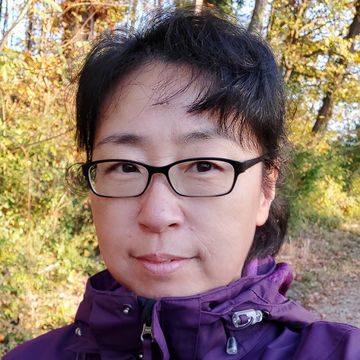# Chair in Applied Analysis

#### Prof. boshi. Li ChenOur current research interests are on “Applied analysis”, mainly focused on partial differential equations and their applications in other sciences such as physics, biology and economics.

Some selected topics are

• Complex systems and their related kinetic equations;
• Population dynamics with diffusion, self-diffusion and cross-diffusion;
• Singular limits in partial differential equations;
• Partial differential equations in semiconductor modelling;
• Fluid-dynamics type systems;
• Stability of matter in quantum mechanics;
• Parabolic Monge-Ampere equation and related curvature flows;
• Derivation of the mean-field limits for interacting particle systems.